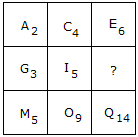# Verbal Reasoning - Character Puzzles - Discussion

### Discussion :: Character Puzzles - Character Puzzles 1 (Q.No.1)

1.

Which one will replace the question mark ?[A]. L10 [B]. K15 [C]. I15 [D]. K8

Explanation:How the number is obtained?

2 + 4 = 6

5 + 9 = 14

Similarly,

3 + 5 = 8

 Srilatha said: (Oct 9, 2010) I did not understand how K8 is the answer. Were as I5 + 2 should be k7.

 Monica said: (Mar 20, 2012) I did not understand how K8 is the answer. Were as I5 + 2 should be k7 ?

 Mukesh said: (Mar 29, 2012) @ Srilatha and Monica You can count number upward to downward i.e. 2+3=5 4+5=9 6+?=14, So ?=14-6 = 8. So, Ans is K8.

 Kandukuri Siva said: (Dec 22, 2012) 1 more possible is 2 4 6 3 5 ? +2 +4 +6 5 9 14 and characters are alternates of alphabets..A_c_E_G_I_K_M_O_Q I hope it help you.

 Subhasmita Dey said: (Nov 27, 2014) It could also be vertically like 2+3 = 5, 4+5 = 9. So 6+? = 14. Hence, 14-6 = 8. Answer remains the same.

 Sakshi Bhingude said: (Oct 29, 2015) It is the most easy question I ever solved. 1st alphabet should be forwarded that much times the number is given below.

 Pranavi said: (Feb 9, 2016) Here alphabet will increase by 2. Like A+2 is C. C+2 is E. And we have given two numbers. I think everyone can understand easily.

 Keshav said: (Oct 22, 2016) I understood this sum it's very easy now. a2 + c4 =e6. 2 + 4 = 6.

 Dilip Mandal said: (Nov 15, 2016) This process is very easy too understand, ignore the alphabet given and only take the sum of letter given. Eg. 2 + 4 = 6. 5 + 9 = 14 then, 3 + 5 = 8, So, K8 is the answer.

 Praveen said: (Apr 16, 2017) ACE one letter difference. GIK one letter difference 246(even)358(odd). So, the answer is k8.

 Shibani Pradhan said: (Jul 20, 2017) Option d is the correct answer. I agree.

 Ravi Shankar said: (Aug 21, 2017) 5, 7, 3. 20, 30, 10. 95, 45, ? Please answer this question.

 Chotu Naz Charak said: (Sep 16, 2017) Simply, Guys just ignore the alphabet and their gap. Just count the digit horizontally and I hope you will get the right answer as K8 comes here.

 Shree said: (May 27, 2018) 95 * 2-45 = 145.

 Nid said: (Jun 14, 2018) @Ravi Shankar. 5+7+3=15=1+5 = 6, 20+30+10=60=6+0 = 6, 95+45+x=23+x=2+3+x = 5+x, At x we need a number. Whose sum is 1 so as to make it 6. Like 55,82,10,100 etc..

 Akshita said: (Jan 18, 2019) I don't understand. Please explain it 2 me.

 Shaiju Simon said: (Mar 1, 2020) A2+C4 = E6. G3+i5 = k8. M5+O9 = Q14.

 Abijit said: (Jul 15, 2020) Didn't understand. Please anyone explain me.

 Armogan said: (Aug 29, 2020) Here the Base numbers of first two alphabets r added to get the third alphabet Base number. A2 + C4 = E6 ,similarly. G3 + I5 = K8 and You can observe the table in which column-wise also the last box contains a Base number which is the sum of above two boxes, One can also get an idea that the middlebox Base number can be obtained by subtracting the first box and last box Base numbers.

 Fbdd said: (Mar 21, 2022) Thanks everyone for explaining the answer.